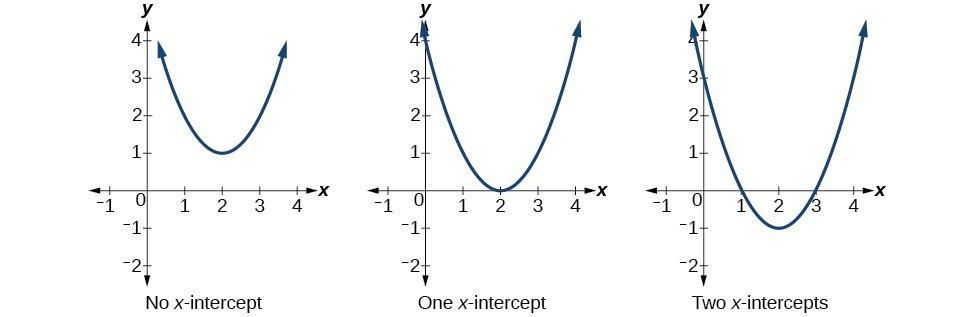### Learning Outcomes

• Find the $y$-intercept of a quadratic function.
• Find the real-number $x$-intercepts, or roots of a quadratic function using factoring and the quadratic formula.

Much as we did in the application problems above, we also need to find intercepts of quadratic equations for graphing parabolas. Recall that we find the $y$-intercept of a quadratic by evaluating the function at an input of zero, and we find the $x$-intercepts at locations where the output is zero. Notice that the number of $x$-intercepts can vary depending upon the location of the graph.Number of $x$-intercepts of a parabola

Mathematicians also define $x$-intercepts as roots of the quadratic function.

### How To: Given a quadratic function $f\left(x\right)$, find the y– and x-intercepts.

1. Evaluate $f\left(0\right)$ to find the $y$-intercept.
2. Solve the quadratic equation $f\left(x\right)=0$ to find the $x$-intercepts.

### Example: Finding the y– and x-Intercepts of a Parabola

Find the $y$– and $x$-intercepts of the quadratic $f\left(x\right)=3{x}^{2}+5x - 2$.

In the above example the quadratic was easily solved by factoring. However, there are many quadratics that cannot be factored. We can solve these quadratics by first rewriting them in standard form.

### How To: Given a quadratic function, find the x-intercepts by rewriting in standard form.

1. Substitute $a$ and $b$ into $h=-\dfrac{b}{2a}$.
2. Substitute $x=h$ into the general form of the quadratic function to find $k$.
3. Rewrite the quadratic in standard form using $h$ and $k$.
4. Solve for when the output of the function will be zero to find the $x$intercepts.

### Example: Finding the Roots of a Parabola

Find the $x$-intercepts of the quadratic function $f\left(x\right)=2{x}^{2}+4x - 4$.

### Try It

Solve ${x}^{2}+x+2=0$.

### Example: Applying the Vertex and x-Intercepts of a Parabola

A ball is thrown upward from the top of a 40 foot high building at a speed of 80 feet per second. The ball’s height above ground can be modeled by the equation $H\left(t\right)=-16{t}^{2}+80t+40$.

a. When does the ball reach the maximum height?

b. What is the maximum height of the ball?

c. When does the ball hit the ground?

### Try It

A rock is thrown upward from the top of a 112-foot high cliff overlooking the ocean at a speed of 96 feet per second. The rock’s height above ocean can be modeled by the equation $H\left(t\right)=-16{t}^{2}+96t+112$.

a. When does the rock reach the maximum height?

b. What is the maximum height of the rock?

c. When does the rock hit the ocean?

## Contribute!

Did you have an idea for improving this content? We’d love your input.